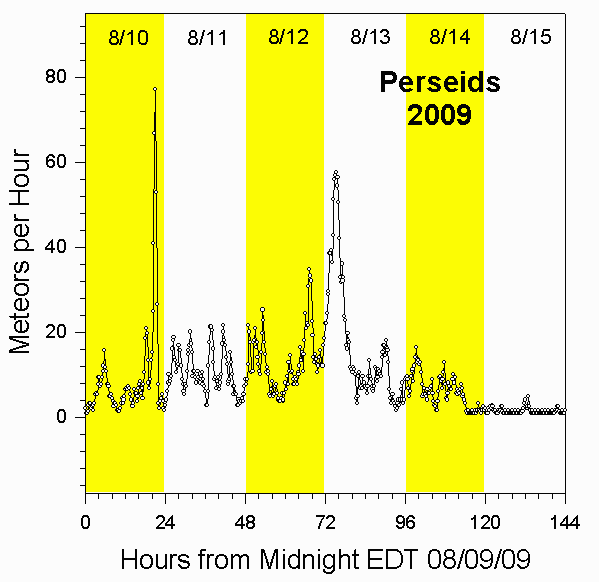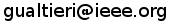Radio Observation of the 2009 Perseids

Radio Observation of the 2009 Perseids

Data Collection and Analysis

For details about our equipment and method of data analysis, see our main page. The 2009 Perseid meteor shower exhibited two radio peaks with counting rates of about 75 meteors per hour around 9:00 PM EDT on August 10, 2009; and 55 meteors per hour around 4:00 AM EDT on August 13, 2009.

In the following summary graph, we've counted meteors in fifteen minute intervals, and then applied a data smoothing equation of the form

Smoothed_Rate(t) = 4*[((1/2)*N(t))+((1/4)*(N(t+1))+((1/4)*(N(t-1))+((1/8)*(N(t+2))+((1/8)*(N(t-2))]

where N(t) is the number of meteors in the fifteen minute interval t, N(t-1)is the number of meteors in the preceding fifteen minute interval, and so on.

Rates for 2009 Perseids

The following graph shows the meteor count rates per hour we measured for the 2009 Perseids. These rates were determined by a computer program that counted all peaks that climbed at least 20% above the background level in one second. The graph shows the rates observed for the six day interval starting at Midnight EDT on 08/09/09.Summary

The 2009 Perseid meteor shower exhibited two radio peaks with counting rates of about 75 meteors per hour around 9:00 PM EDT on August 10, 2009; and 55 meteors per hour around 4:00 AM EDT on August 13, 2009.

 Comments and technical questions can be addressed to